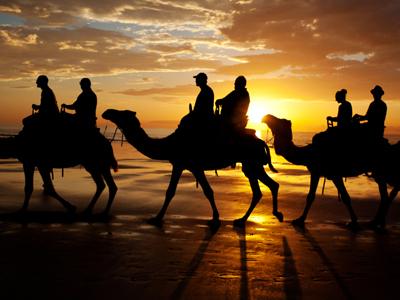Camels have big feet so they have a large area and the pressure is small. This means they don't sink into soft sand.

# Pressure 02

## Revise what you know about the equation for working out pressure. What is a Fat Bike?

### Scroll down to play the quiz

Test your knowledge of pressure in this KS3 Science quiz. Pressure is measured in Pascals. A pascal is a very small unit which is equal to spreading the weight of a small-sized apple over an area of one square metre. You can see a knowledge of pressure in action almost every day. Tractors have large wide tyres so they put less pressure on the soil in the fields - crops don't grow as well if the soil is compacted. Some mountain bikes, called 'fat bikes', have much wider tyres than normal mountain bikes. These put less pressure on the ground and can even be ridden on snow!

The equation for working out the pressure is to divide the force by the area on which it is acting. Questions about pressure will also require you to change the equation round to work out the force or the area when you are given the other two numbers. To calculate the area a force acts on to produce a pressure, you need to use the following equation: area = force/pressure and to work out the force from a pressure acting on a certain area: force = pressure x area.

1.
A box weighs 100 N. It exerts a pressure of 25 Pa on a table. What is the area of the box in contact with the table?
4 mm2
4 cm2
4 m2
4 km2
Area = force/pressure = 100/25. Remember weight is a force
2.
A box weighs 20 N. It exerts a pressure of 2 Pa on a table. What is the area of the box in contact with the table?
10 cm2
0.1 m2
10 m2
40 m2
Area = force/pressure = 20/2 = 10 m2
3.
A skier weighs 600 N and exerts a pressure of 2,500 Pa on the snow. What is the area of the skis?
0.12 m2
0.24 m2
1.2 m2
4.2 m2
Area = 600/2,500 = 0.24 m2
4.
A skier weighs 600 N, lifts one ski up and exerts a pressure of 5,000 Pa on the snow. What is the area of one of the skis?
0.12 m2
0.24 m2
0.48 m2
1.2 m2
Area = 600/5,000
5.
A student weighs 500 N, standing on two feet she exerts a pressure of 25,000 Pa on the ground. What is the area of one foot?
50 cm2
0.005 m2
0.01 m2
0.02 m2
Area = 500/25,000 = 0.02 (two feet), one foot = 0.01 m2
6.
One box weighing 60 N is put on top of one weighing 120 N. The pressure they exert is 180 Pa. What is the area touching the ground?
0.67 m2
1 m2
2 m2
3 m2
Area = (60 + 120)/180
7.
A 60,000N elephant can stand on one foot! The pressure it exerts is 240,000 Pa. What is the area of its foot?
0.16 m2
0.24 m2
0.25 m2
4 m2
Area = 60,000/240,000
8.
Camels have big feet so they don't sink into soft sand because .......
they have a large area so the pressure is large
they have a large area so the pressure is small
they have a small area so the pressure is large
they have a small area so the pressure is small
Spreading their weight out over as large an area as possible means they can walk more easily over soft sand
9.
A sharp knife cuts well because .......
it has a large area so pressure is large
it has a large area so pressure is small
it has a small area so pressure is large
it has a small area so pressure is small
A sharp blade is sharp because it has been ground to a thin edge with a very small area
10.
Doubling the force and doubling the pressure mean .......
that area doubles
that area gets larger
that area gets smaller
that area stays the same
Area = force/pressure so if you double both force and the pressure, the area over which the force is applied will remain the same